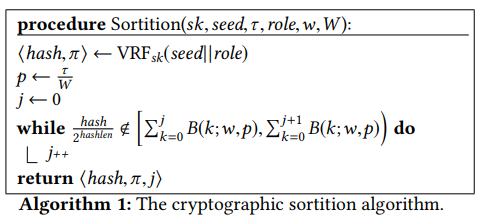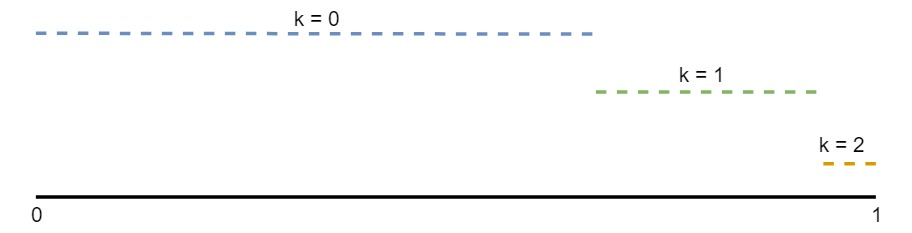# The intuition behind Algorand Cryptographic Sortition

An essential part of Algorand consensus mechanism is the committee election. This election is somewhat counterintuitive since every user runs the code that could decide if it is elected; this is called cryptographic sortition.

At first, this may sound like a bad idea since delegating to every user the execution of the decision seems too risky. However, if it is correctly designed to make cheating infeasible, it may be an exciting idea, i.e., not depending on trusted parties to committee election. Additionally, since executions can be parallelized by users and do not require interaction, it may be quite fast (in Algorand’s case, it is).

If this is not the first time you hear a crazy idea in the blockchain space, you are probably betting that mathematics is behind the scenes. Guess what? You are right. However, can we have some practical intuition about how this works?

If we dig into this paper we can find the exact description of how Algorand runs the sortition:White paper — Page 55

Ok, this may seem intimidating but hold on a second.

This algorithm has two main components:

• A call to a VRF function.
• Do some things that depend on that result.

The goal of this article is to explain the latter. Understanding what VRF does and how it works is a critical part of the sortition. Most of the fairness and security of the sortition depends on it. Sergey, head of cryptography at Algorand, has already made an article to explain how the VRF works, here. You can read it now or later since we are going to assume that it works correctly.

To abstract our analysis from the VRF mechanics, let’s assume the following two sentences to be true. The VRF function gives a unique and verifiable random number depending on the three variables involved. Any other party can verify that this random number was generated by the function and corresponds to the public key of the user who generated it.

If you remind a bit of combinatorics, you can have some intuition of what is going on on the while loop, but I’ll try to make a bottom-up approach to explaining what is going on here.

For the moment, forget the algorithm and let’s do a multi-step mental exercise. Hopefully, in the end, we’d see that the algorithm isn’t obscure and makes much sense.

#### Step 1 — Place all the existing coins on a table

We know that there are a total of W coins in the blockchain, so we imagine them placed on a table one next to each other.

#### Step 2 — A simple repeated experiment

To continue, I give you a button with a screen. Every time you hit this button the screen have one of two outputs: success, or failure. The output of hitting this button is probabilistic, where success has a probability p=t/W and failure (1–p), t is a parameter of the system. Every button hit output doesn’t depend on the result obtained in previous hits. You can think of this button as flipping a biased coin.

Now you take the first coin in the table and hit the button. You label the coin with the result of the output screen. Next, you do the same with the second coin. Next, again the same with the third, fourth, until you finish labeling all W coins.

Let’s pause for the moment and think about what we’ve done. The expected result of doing this experiment is having roughly W*p coins labeled as success. Since the button output has a success probability p if we hit it W times, it’s pretty natural to expect to have W*p successes. With the same logic, tossing 100 times a fair coin (p=0.5) results, roughly, in 50 heads and 50 tails.

Since p=t/W, this means that W*p is close to t. So, we can parametrize t conveniently to control which is the expected amount of coins that are labeled success. In particular, in Algorand this is tuned to control the committee-size.

#### Step 3 — Coins have owners, but that doesn’t change anything!

A user owns every coin in Algorand. Imagine user U1 owns the first M1 coins, user U2 owns M2, and so on.

It’s pretty clear that the experiment we did in Step 2 will have the same result we saw before since coin ownership doesn’t change anything in the experiment. However, now we can interpret the result of the experiment from another angle. We can focus on how many coins were labeled success in each user. Also, it’s pretty clear that the more coins a user has, we’d expect to have more success coins.

#### Step 4 — How many successes had each user?

If a user has w coins, we could be interested in what is the probability that he/she would have 0 coins labeled as success, or 1, 2 , up to w coins. Since each coin labeling is independent of each other, this matches the exact definition of a binomial distribution.

Each button hit is a Bernoulli trial with probability p. If we define X as a random variable representing how many of the w coins were labeled as success, then X ~ Binomial(w, p), i.e. probability of successes of multiple independent Bernoulli trials. Thus, P(X=k)=B(k, w, p).

#### Step 5 — Visualize each probability

If a user with w coins runs this experiment multiple times, it’s pretty clear that the amount of possible success range between 0 and w. More formally, P(X=0)+P(X=1)+…+P(X=w)=1.

What I think helps a lot in understanding the algorithm is visualization. Let’s assume w, the total coins of our user is two:Sketch of B(k,2,p)

Since W, total coins in the blockchain, is very big we know that p is tiny. Since p is so small, if we run the experiment in each of our two coins the most probable output, by far, will be that none of them are labeled success. Being luckier, we would see that only one of them was labeled success. Moreover, if we were fortunate, the two of them were labeled success.

Now imagine you randomly throw a dart in this segment [0,1]. It’s pretty clear that the probability of this number lying in the segment k, corresponds to the length of this segment, B(k,w,p). Thus, this is the same as running an execution of the experiment!

Saying it differently, throwing a random dart in [0,1] is like sampling from the random variable X.

More generally for the mathematically inclined, you can also imagine sampling from a distribution by using its cumulative probability function. You throw a dart on the Y axis, and then project to the function value; the corresponding X value is your sampled output.

#### Step 6 — This is the while loop!

The while loop in the algorithm is doing precisely that!

Given a random point hash/pow(2, hashlen) in [0,1], the loop finds out in which segment does the random point lies. Depending on which segment contains the point, we can say that the sampled X is j.

So this algorithm merely is simulating how many of the coins of a user won a lottery. Which lottery? A lottery where all the coins in the system participate, and where each coin has probability p of being success, with some probability p that will result in expected W*p successes.

### Where does the VRF fit here?

A bigger j means that the user has more voting power in the committee. A malicious user is interested in hash/pow(2, hashlen) to be as big as possible, thus, try to generate the biggest hash possible.

Unfortunately for him, the hash is the result of the VRF function which depends on sk, seed, and role. This means that he can’t invent the hash value and convince others that the hash is correct; he needs proof returned by the VRF to persuade others.

What about manipulating the three parameters to gain a little advantage? The role value doesn’t give us any room for exploring multiple hashes. The seed is a system wide value. The white paper discusses how seed is generated in section 5.2.

His last hope is trying with multiple sk to influence the result, but no luck here. The consensus algorithm forces sk to be generated before the seed was agreed; you can find more about this in section 5.3 or Sergey’s post.

### Sybil attacks

A malicious user could think that pretending being multiple distinct users may give him an advantage, but since each experiment in the coins is independent of each other, it’s not the case.

Imagine a malicious user has 100 coins and runs our experiment having a total of 5 coins labeled success. This fact doesn’t change if he pretended that 20 of the coins were for some user U1 and the other 80 for other user U2. Since voting power only depends on the number of coins, splitting coin ownership in multiple users is worthless.

For the mathematically inclined, if X~Binomial(k1,p) and Y~Binomial(k2,p), then X+Y~Binomial(k1+k2,p).

An important detail is that splitting the coins into two different users guarantees independence in the experiments. Again, the VRF function has the responsibility of making this guarantee to avoid the malicious user breaking this assumption.

### Conclusion

In this article, we explored a bottom-up approach to understand cryptographic sortition which, hopefully, helps to understand better an essential part of Algorand blockchain lifecycle.

We also analyzed possible attack vectors that might try to influence the result of the sortition with the goal of having more voting power in the next round of block election.

My primary interest as an Algorand Ambassador is to explain the technologies behind Algorand to the biggest possible audience. You’re invited to join the growing community in Discourse and on Telegram too.